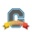cancel
Showing results for
Did you mean:Surveyor

## Scientific Notation

How can I create a question in canvas quiz where students can give their answer in
scientific notation?
This is a very basic functionality, but I cant figure out how to do it in canvas.

Tags (1)
1 Solution

Accepted SolutionsLearner II

I got around this by making students enter the coefficient and the power of 10 using drop down boxes.  Here is a screen grab of what that looks like.8 RepliesCommunity Coach

@epolomsk , here a conversation about scientific notation that might help - https://community.canvaslms.com/message/131133-scientific-notation-in

KonaSurveyor

hmm that doesnt help. I want t he students to type scientific notation. That old post doesnt explain how to do that.
It states that the browser converts enotation to decimal.

I want to give students a decimal and have them write scientific notation.Surveyor

Supposedly you can do this with a numeric answer with Precision.  But, I cannot get it to work.  Even with precision of 10 or 16, it was rounding 1.45e-5 to zero.  Very frustrating.Surveyor

Using E notation used to work, even as recently as June.  Some thing has changed to make it not work, and only accept numerical answers.Surveyor

It's a bug.  They added some kind of verification filter to quizzes for numeric and formula answers to prevent folks from typing in letters and the filter doesn't recognize the "e" in 6.022e+23 as part of a number.  Apparently they have a fix, but it hasn't been pushed to the live servers yet. Not sure why.  Might be part of a larger bug fix that requires other things to be ready for it to go out.Learner II

I got around this by making students enter the coefficient and the power of 10 using drop down boxes.  Here is a screen grab of what that looks like.Surveyor

This was a major frustration last spring (2020), but there's some good news...  the "NEW QUIZ" feature (not available everywhere) lets you generate formula questions and accept answers in scientific notation.

It's inflexible,

Let's say the answer in non-sci notation is 1234.56

if you require (e.g.) 2 decimal places in the answer, you would have to answer 1.23*10^3.   1.234*10^3 would be rejected.  Other rejected answers include 1.23*10^03, 1.23E3, 1.23e3, you get the idea.  just x.xx * 10^y

It's ironic that the generated answers appear to the instructor as 1.23 x 10^3, but that would be rejected as a correct answer.  It has to be 1.23 * 10^3.

HOWEVER, you can specify a RANGE of correct answers using sci notation.  For example, the sort(1000) = 31.62, and you can set it to accept anything between 3.10*10^1 and 3.20*10^1.  It auto graded correctly, giving credit for 31.62, 3.12, and 3.29,

I had to specifically request to add the "New Quiz" option to my courses, but I think it's worth it.Surveyor

Oops, some typos in my last answer.  Here's the corrected last paragraph.

HOWEVER, you can specify a RANGE of correct answers using sci notation.  For example, the sqrt(1000) = 31.62, and you can set it to accept anything between 3.10*10^1 and 3.20*10^1.  It auto graded correctly, giving credit for 3.162*10^1, 3.11*10^1, and 3.19*10^1.